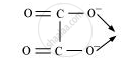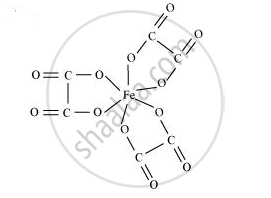Share

# Amongst the Following, the Most Stable Complex is - CBSE (Science) Class 12 - Chemistry

ConceptImportance and Applications of Coordination Compounds

#### Question

Amongst the following, the most stable complex is

(i) [Fe(H2O)6]3+

(ii) [Fe(NH3)6]3+

(iii) [Fe(C2O4)3]3−

(iv) [FeCl6]3−

#### Solution 1

We know that the stability of a complex increases by chelation. Therefore, the most stable complex is [Fe(C2O4)3]3−.Then#### Solution 2

In each of the given complex, Fe is in + 3 oxidation state. As C2042-is didentate chelating ligand, it forms chelate rings and hence (iii) out of complexes given above is the most stable complex.

Is there an error in this question or solution?

#### APPEARS IN

NCERT Solution for Chemistry Textbook for Class 12 (2018 to Current)
Chapter 9: Coordinate Compounds
Q: 31 | Page no. 260

#### Video TutorialsVIEW ALL 

Solution Amongst the Following, the Most Stable Complex is Concept: Importance and Applications of Coordination Compounds.
S